Latest Banking jobs   »

# Reasoning Ability Quiz For FCI Phase I 2022- 15th September

Direction (1-5): In these questions, relationship between different elements is show in the statements. The statements are followed by conclusions. Study the conclusions based on the given statements and select the appropriate answer:
(a) If only conclusion I follows.
(b) If only conclusion II follows.
(c) If either conclusion I or II follows
(d) If neither conclusion I nor II follows.
(e) If both conclusions I and II follow.

Q1. Statements: P>O, K≥M>T, O=N<J, R<T, J>K
Conclusion I: T≤N
II: N<T

Q2. Statements: T=Y, D<R, U≤Z, X>B>O, Y<R≤U<O
Conclusion I: B>D
II: Z>R

Q3. Statements: H=I≤S, Y=N, E=L<M, N≥S≥E
Conclusion I: Y>L
II: Y=L

Q4. Statements: P<V<X, E>H, R≥O≥L, H>V<L
Conclusion I: P<R
II: E>P

Q5. Statements: R>T=B, H≥D<S, L≥I=P, L<S<B
Conclusion I: R>P
II: H>S

Directions (6-10): In these questions, relationship between different elements is shown in the statements. These statements are followed by some conclusions. Read the statements and then decide which of the following conclusions follow from the given statements.

Q6. Statement: F<T≤N, F>S, M≤T<G
Conclusions: I. F<N
II. G>T
III. M≤N
IV. F=M
(a) Only I and II follow
(b) Only III and IV follow
(c) Only I, II and III follow
(d) Only I and IV follow
(e) All follow

Q7. Statement: L≥K<E≥A>F≥B
Conclusions: I. F<E
II. B≤E
III. K>F
IV. L>A
(a) Only I follow
(b) Only II and IV follow
(c) Only III and IV follow
(d) Only II follows
(e) Only I and III follow

Q8. Statement: U≥V>C, Y≤J>C
Conclusions: I. U>J
II. Y>V
III. U>C
IV. V<J
(a) Only I and III follow
(b) Only II follows
(c) Only III follows
(d) Only II and IV follow
(e) Only I and IV follow

Q9. Statement: D≥H>K=X≥Q, K>S≥T
Conclusions: I. X>T
II. S≤Q
III. D>T
IV. H>S
(a) Only I and II follow
(b) Only II and III follow
(c) Only III and IV follow
(d) Only I, III and IV follow
(e) Only II and IV follow

Q10. Statement: I>O≥P, E<R>I≥T
Conclusions: I. R>O
II. E>T
III. P<R
IV. O>T
(a) Only I and III follow
(b) Only III and IV follow
(c) Only I, II and III follow
(d) Only I and IV follow
(e) All follow

Q11. In which of the following expressions will the expression. ‘W<Z’ Definitely true?
(a) W>Y≥X<Z≤V
(b) W≤Y=V<Z≤X
(c) Y≤W>X<V≤Z
(d) Z≤Y<W>X=V
(e) None of these

Q12. if the given expression ‘P<Q≥R>T=S’ is definitely true, then which of the following expressions will be true?
(a) P<S
(b) T≥R
(c) Q≤S
(d) P<R
(e) Q>T

Q13. If the expressions, ‘J>L≤K<M’,’N≥K’ and ‘L≥O’ are true then which of the following combinations will be definitely true?
(a) K ≥ O
(b) J < O
(c) N = O
(d) J > M
(e) None of these

Q14. In which of these expression ‘O > Z’ is definitely True?
(a) L < Z ≥ S ≥ Q < P> A ≥ O > V
(b) P > O > M = D ≥ B = A > Z = R
(c) N ≤ A > O > L ≥ V ≤ B = Z < S
(d) S > O = C ≥ H = H ≥ Z ≤ Q = T
(e) B > O ≤ A = N < Q ≤ T = Z < G

Q15. In which of these expression ‘B ≤ X’ is definitely true?
(a) W < X ≥ S ≥ Q < N> B ≥ R > V
(b) N > B > M = D ≥ Y = L > X = R
(c) M ≤ B > L > W ≥ V ≤ Y = X < S
(d) S > B = C ≤ H = X ≤ Q = T = K
(e) Y > L ≤ B > M ≥ Q < T > X < G

Solutions

S1. Ans. (c)
Sol. I: T≤N(False)
II: N<T (False)

S2. Ans. (a)
Sol. I: B>D (True)
II: Z>R (False)

S3. Ans. (c)
Sol. I: Y>L (False)
II: Y=L (False)

S4. Ans. (e)
Sol. I: P<R (True)
II: E>P (True)

S5. Ans. (a)
Sol. I: R>P (True)
II: H>S (False)

S6. Ans. (c)
Sol. I. F<N(True)
II. G>T(True)
III. M≤N(True)
IV.F=M(False)

S7. Ans. (a)
Sol. I. F<E(True)
II. B≤E(False)
III. K>F (False)
IV. L>A(False)

S8. Ans. (c)
Sol. I. U>J(False)
II. Y>V(False)
III. U>C (True)
IV. V<J(False)

S9. Ans. (d)
Sol. I. X>T (True)
II. S≤Q(False)
III. D>T (True)
IV. H>S(True)

S10. Ans. (a)
Sol. I. R>O (True)
II. E>T(False)
III. P<R (True)
IV. O>T(False)

S11. Ans. (b)
Sol. W≤Y=V<Z≤X

S12. Ans. (e)
Sol. Q ≥ R > T

S13. Ans. (a)
Sol. O ≤ L ≤K

S14. Ans. (b)

S15. Ans. (d)#### Congratulations!Download Hindu Review of October 2021: Free PDFIncorrect details? Fill the form again here

Download Hindu Review of October 2021: Free PDF

Thank You, Your details have been submitted we will get back to you.
•World Teacher's Day 2022, Theme, History...
•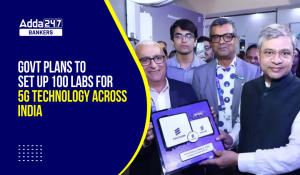Govt plans to set up 100 labs for 5G tec...
•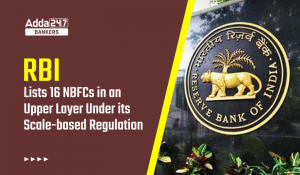RBI Lists 16 NBFCs in Upper Layer Under ...
•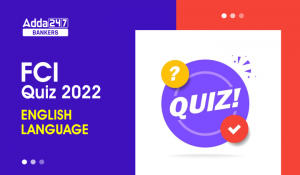English Quizzes For FCI Phase 1 2022- 3r...
•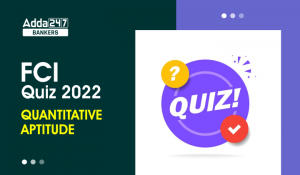Quantitative Aptitude Quiz For FCI Phase...
•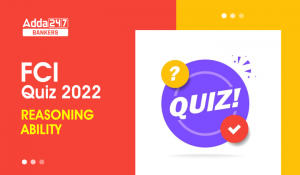Reasoning Ability Quiz For FCI Phase I 2...